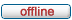It is currently Sun May 31, 2020 10:05 am

 All times are UTC

 Print view Previous topic | Next topic
Author Message
 Post subject: bset/bclr corrupting memory with 32-bit addressesPosted: Thu Aug 06, 2015 4:13 pmJoined: Thu Aug 06, 2015 12:18 am
Posts: 1
When used with full 32-bit addresses (that is, non-zero data in the highest 8 bits), the bset and bclr instructions will frequently either corrupt data at the specified address or generate a runtime exception.

Attached is sample code that demonstrates this behavior as well as a runtime log. Note that, at the end of execution, the value of the byte at \$FF2000 is \$79. If the simulator were operating correctly, the expected value would be \$0C.

I suspect that the simulator is not correctly dropping the high byte of the address, and that this issue also affect the bchg instruction. However, I have not personally tested anything other than bset and bclr.

(Apologies for the large copy/paste. The forum's attachment system will not allow me to upload .X68 or .txt files.)

Sample code:
Code:
ORG    \$1000

TestVar equ \$FFFF2000

START:
lea TestVar, a0
move.b #0, (a0)

bclr #0, (a0)

bset #2, (a0)
bset #3, (a0)

SIMHALT

END START

*~Font name~Courier New~
*~Font size~10~
*~Tab type~1~
*~Tab size~4~

Runtime log:
Code:
EASy68K execution log file: 8/5/2015 3:51:14 PM

00FF2000: FF FF FF FF FF FF FF FF FF FF FF FF FF FF FF FF ----------------

D0=00000000 D4=00000000 A0=00000000 A4=00000000    T_S__INT___XNZVC
D1=00000000 D5=00000000 A1=00000000 A5=00000000 SR=0010000000000000
D2=00000000 D6=00000000 A2=00000000 A6=00000000 US=00FF0000
D3=00000000 D7=00000000 A3=00000000 A7=01000000 SS=01000000
PC=00001000  Code=41F9 FFFF2000          Line=    6      lea TestVar, a0

00FF2000: FF FF FF FF FF FF FF FF FF FF FF FF FF FF FF FF ----------------

D0=00000000 D4=00000000 A0=FFFF2000 A4=00000000    T_S__INT___XNZVC
D1=00000000 D5=00000000 A1=00000000 A5=00000000 SR=0010000000000000
D2=00000000 D6=00000000 A2=00000000 A6=00000000 US=00FF0000
D3=00000000 D7=00000000 A3=00000000 A7=01000000 SS=01000000
PC=00001006  Code=10BC 0000              Line=    7      move.b #0, (a0)

00FF2000: 00 FF FF FF FF FF FF FF FF FF FF FF FF FF FF FF ----------------

D0=00000000 D4=00000000 A0=FFFF2000 A4=00000000    T_S__INT___XNZVC
D1=00000000 D5=00000000 A1=00000000 A5=00000000 SR=0010000000000100
D2=00000000 D6=00000000 A2=00000000 A6=00000000 US=00FF0000
D3=00000000 D7=00000000 A3=00000000 A7=01000000 SS=01000000
PC=0000100A  Code=0890 0000              Line=    9      bclr #0, (a0)

00FF2000: 70 FF FF FF FF FF FF FF FF FF FF FF FF FF FF FF p---------------

D0=00000000 D4=00000000 A0=FFFF2000 A4=00000000    T_S__INT___XNZVC
D1=00000000 D5=00000000 A1=00000000 A5=00000000 SR=0010000000000100
D2=00000000 D6=00000000 A2=00000000 A6=00000000 US=00FF0000
D3=00000000 D7=00000000 A3=00000000 A7=01000000 SS=01000000
PC=0000100E  Code=08D0 0002              Line=   11      bset #2, (a0)

00FF2000: 75 FF FF FF FF FF FF FF FF FF FF FF FF FF FF FF u---------------

D0=00000000 D4=00000000 A0=FFFF2000 A4=00000000    T_S__INT___XNZVC
D1=00000000 D5=00000000 A1=00000000 A5=00000000 SR=0010000000000100
D2=00000000 D6=00000000 A2=00000000 A6=00000000 US=00FF0000
D3=00000000 D7=00000000 A3=00000000 A7=01000000 SS=01000000
PC=00001012  Code=08D0 0003              Line=   12      bset #3, (a0)

00FF2000: 79 FF FF FF FF FF FF FF FF FF FF FF FF FF FF FF y---------------

D0=00000000 D4=00000000 A0=FFFF2000 A4=00000000    T_S__INT___XNZVC
D1=00000000 D5=00000000 A1=00000000 A5=00000000 SR=0010000000000100
D2=00000000 D6=00000000 A2=00000000 A6=00000000 US=00FF0000
D3=00000000 D7=00000000 A3=00000000 A7=01000000 SS=01000000
PC=00001016  Code=FFFF FFFF              Line=   14      SIMHALT

TopPosted: Thu Aug 13, 2015 1:28 pmJoined: Thu Dec 16, 2004 6:42 pm
Posts: 1122
Corrected in Sim68K v5.15.04

_________________
Prof. Kelly

TopDisplay posts from previous: All posts1 day7 days2 weeks1 month3 months6 months1 year Sort by AuthorPost timeSubject AscendingDescending

 All times are UTC

#### Who is online

Users browsing this forum: No registered users and 4 guests

 You cannot post new topics in this forumYou cannot reply to topics in this forumYou cannot edit your posts in this forumYou cannot delete your posts in this forumYou cannot post attachments in this forum

Search for:
 Jump to:  Select a forum ------------------ EASy68K Forum    FAQ    Latest Features    EASy68K Questions    68000 Programming Questions    Wish List    Wishes Granted    Undocumented Features    Documented Features    General Discussion    EASy68K Projects